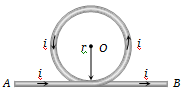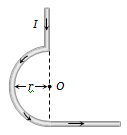The earth’s magnetic field at a given point is $0.5×{10}^{-5}Wb-{m}^{-2}.$ This field is to be annulled by magnetic induction at the center of a circular conducting loop of radius 5.0cm. The current required to be flown in the loop is nearly :

(a) 0.2 A                   (b) 0.4A

(c) 4A                       (d) 40A

Concept Questions :-

Magnetic field due to various cases
High Yielding Test Series + Question Bank - NEET 2020

Difficulty Level:

A part of a long wire carrying a current i is bent into a circle of radius r as shown in figure. The net magnetic field at the centre O of the circular loop is(a) $\frac{{\mu }_{0}i}{4r}$                                 (c) $\frac{{\mu }_{0}i}{2\mathrm{\pi r}}\left(\mathrm{\pi }+1\right)$

(b) $\frac{{\mu }_{0}i}{2r}$                                 (d) $\frac{{\mu }_{0}i}{2\mathrm{\pi r}}\left(\mathrm{\pi }-1\right)$

Concept Questions :-

Magnetic field due to various cases
High Yielding Test Series + Question Bank - NEET 2020

Difficulty Level:

In the figure, what is the magnectic field at the point O(a) $\frac{{\mu }_{0}I}{4\mathrm{\pi r}}$                                   (c) $\frac{{\mu }_{0}I}{4r}+\frac{{\mu }_{0}I}{4\mathrm{\pi r}}$

(b) $\frac{{\mu }_{0}I}{4\mathrm{\pi r}}+\frac{{\mu }_{0}I}{2\mathrm{\pi r}}$                         (d) $\frac{{\mu }_{0}I}{4r}-\frac{{\mu }_{0}I}{4\mathrm{\pi r}}$

Concept Questions :-

Magnetic field due to various cases
High Yielding Test Series + Question Bank - NEET 2020

Difficulty Level:

The magnetic moment of a current (i) carrying circular coil of radius (r) and number of turns (n) varies as :

(a) $1/{r}^{2}$                             (b) $1/r$

(c) r                                   (d) ${r}^{2}$

Concept Questions :-

Magnetic moment
High Yielding Test Series + Question Bank - NEET 2020

Difficulty Level:

The current is flowing in the south direction along a power line. The direction of the magnetic field above the power line (neglecting earth's field) is :

(a) South                         (b) East

(c) North                          (d) West

Concept Questions :-

Magnetic field due to various cases
High Yielding Test Series + Question Bank - NEET 2020

Difficulty Level:

For the magnetic field to be maximum due to a small element of current carrying conductor at a point, the angle between the element and the line joining the element to the given point must be :

(a) 0°                            (b) 90°

(c) 180°                         (d) 45°

Concept Questions :-

Biot-Savart law
High Yielding Test Series + Question Bank - NEET 2020

Difficulty Level:

An electron and a proton enter a magnetic field perpendicularly. Both have the same kinetic energy. Which of the following is true:

1. Trajectory of electron is less curved

2. Trajectory of proton is less curved

3. Both trajectories are equally curved

4. Both move on a straight-line path

Concept Questions :-

Lorentz force
High Yielding Test Series + Question Bank - NEET 2020

Difficulty Level:

A proton, a deuteron, and an $\alpha -$ particle having the same kinetic energy are moving in circular trajectories in a constant magnetic field. If ${r}_{p},{r}_{d}$ and ${r}_{\alpha }$ denote respectively the radii of the trajectories of these particles, then:

1. ${r}_{\alpha }={r}_{p}<{r}_{d}$

2.  ${r}_{a}>{r}_{d}>{r}_{p}$

3. ${r}_{\alpha }={r}_{d}>{r}_{p}$

4.  ${r}_{p}={r}_{d}={r}_{\alpha }$

Concept Questions :-

Lorentz force
High Yielding Test Series + Question Bank - NEET 2020

Difficulty Level:

An electron and a proton with equal momentum enter perpendicularly into a uniform magnetic field, then :

(a) The path of proton shall be more curved than that of electron

(b) The path of proton shall be less curved than that of electron

(c) Both are equally curved

(d) Path of both will be a straight line

Concept Questions :-

Lorentz force
High Yielding Test Series + Question Bank - NEET 2020

Difficulty Level:

One proton beam enters a magnetic field of ${10}^{-4}T$ normally, Specific charge = ${10}^{11}C/kg.$ velocity = ${10}^{7}m/s.$ What is the radius of the circle described by it:

1. $0.1m$

2. $1m$

3. $10m$

4. None of these

Concept Questions :-

Lorentz force